Rigid Body Transformations

To start with basics of robotics we should first know what is a frame in 2D/3D world. A frame is nothing but a coordinate axis attached to a body as shown in below figures.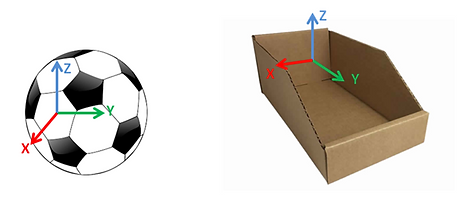Fig. 1 3D Frames attached to objectsFig. 2 2D Frames attached to objects

Key Point: Generally color code is followed for frame representation i.e., Red for X axis, Green for Y axis and  Blue for Z axis. RGB for XYZ

Now, why do we need to attach a frame to a body or what is the use of these frames?

Well the answer is simple, to locate a object's position and orientation with respect to other. An example is illustrated in fig 3.

But how are we going to determine position and orientation of the objects using frames?Fig. 3 Illustrating the Transformation between mouth of the hungry Lion and Bu*t of the scared man

Transformations:

Transformation is simply the change of position and orientation of a frame attached to a body with respect to a frame attached to another body. Transformations in a planar space is known as 2D transformation and transformations in a spatial world is known as 3D transformation

Translation: Change in position

Rotation: Change in orientation

Transformation: Translation + Rotation

2D Transformations:

Let's start with the easy one first, 2D Transformation. We all know that 2D is nothing but a plane which is parallel to two axes and perpendicular to the third axisFig. 4 A XY plane perpendicular to Z-axis

Now a quick question for you,

Q: Let us say you have a toy car on the floor as shown in fig 5. Now how many variables you need, to position this car on the floor?

As the name says 2D transform, so you have guessed 2 variables x,y then you are absolutely wrong. If you guessed 3 variables as x, y and z then you are wrong again but close to the answer.

If (you have guessed it correct):

Congratulations....!!!

else:

You have learnt a new thing.Fig. 5 A toy car placed on floor (Planar Space)

Now let's see how to determine the position and orientation of a  body using frames and 2D Transformation in a plane using above car example.

Wait-a-minute, from which point are we trying to locate the car or with respect to what..?

For that we have to first attach a fixed frame to the floor. This frame is called the "reference frame". Also we have to attach a frame to car.

Let us name this reference frame attached to the floor as "world" frame and frame attached to car as "target" frame.

I will put the world frame in the centre of the floor with X and Y axes parallel to the floor and Z axis coming out of the plane (Use right hand rule to determine third axis).

Let us assume initially both target frame and world frame are in the same position and orientation as shown in the fig 6.

Therefore, the position of target frame (car) with respect to the world frame (observation point) is 0m in X direction and 0m in Y direction (0m,0m). Also the angle of the target frame with respect to fixed to world frame is 0 degrees. Hence the 2D transformation is as below,Fig. 6 Car frame is in same position and orientation w.r.t to floor frameNow I moved the car to a new position from the reference frame. Let's say 1m in X direction and 1m in Y direction and I will not disturb the orientation of the car as shown in the below fig 7.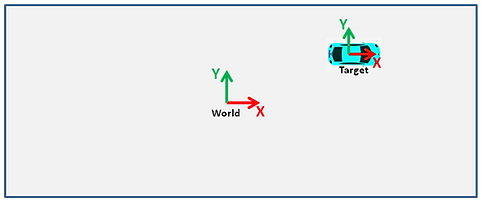This transformation is given as below,Fig. 7

Then I moved the car such that it is 1m far from reference frame along X direction and 0m along Y direction. This time I will rotate the car +45 degrees (use right hand thumb rule to determine +ve or -ve rotation) with respect to the reference frame.  The position of the car will be as shown in fig 8 and the transformation is as below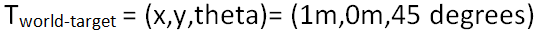Fig. 8

Hope you are clear with 2D transformation and frames with above examples.

Let's get into some real math. Now we will learn how to represent 2D transform in matrix form.

Before that we need to learn about matrix representation of 2D Rotation.

2D Rotation matrix is a 2X2 matrix. It is given byHere θ is angle of rotation.

Now let's build 2D Transformation matrix.

Assume reference frame to be frame A and target frame to be B. Transformation of frame B with respect to frame A is given as below.Here PAB is a 1X2 matrix which represents the translation of frame B with respect to frame A.RAB is rotation matrix which is same as R matrix given above

As you may have noticed, we have added 0's and 1 in the last row of TAB matrix  to simplify matrix operations.

Therefore, 2D transform matrix is a 3X3 matrix and its expanded form is as below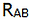Fig. 9 Transformation of frame B w.r.t frame A

Yes..!!! This is how 2D transform matrix looks like.

Now you may be thinking why we are taking pain to represent simple translation and rotation (simple θ) in such a matrix form.

After going through the next example you will get to know how useful it is to represent transformation in a matrix form.Fig. 10 Cars A, B and C in parking Lot

Assume there are three cars in a parking area A, B and C as shown in fig 10.

Person in car A can see Car B but not car C because there is wall between A and C. Person in car B can see car C.

Now let's attach frames to these cars.

Lets say I am sitting in Car A. Since I can see car B, I know the position and orientation of car B with respect to my car. I have figured out that car B is at  [x1,y1] units and rotated at an angle θ1 w.r.t my car.

Hence I can write the transformation matrix TAB as below,Similarly person in car B has figured out that car C is at [x2,y2] units and rotated at an angle θ2 with respect to his car B.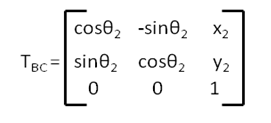Now my task is to find out the position and orientation of car C without seeing it directly. Let's say car C is at [x3,y3] units and rotated at an angle θ3 with respect to my car, car A.

To find our x3,y3 and  θ3, I will take the help of person in car B to get TBC matrix. Therefore I know TAB and TBC. That's it I have all required information to calculate x3,y3 and  θ3.

Now I can derive matrix TAC simply by multiplying TAB and TBC.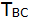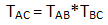Fig. 11 Transformation of Car C w.rt Car A with the help of Car B

From now on I will write cosθ and sinθ in short forms like below

cosθ1: c1, sinθ1 : s1 ; cosθ2: c2, sinθ2: s2 ; cosθ3: c3, sinθ3: s3 ; cosθ: c,sinθ: s

cosθ1* sinθ1 =c1s1  ;  cosθ1* sinθ2 =c1s2  ;  cosθ2* sinθ1 =c2s1 ; so on

Hence the above expression becomes as below,From above equation I can derive x3 and y3 by equating last column elements,Coming to the orientation part i.e., θ3,  I will divide second in first row with second element in the second row,Key Point: The other way to find the Final Rotation in 2D is  just by adding all the rotations. In above example you can find θ3 by adding θ1 and θ2 i.e., θ3= θ1 +θ2. (Bear in mind this way doesn't work in case of 3D rotations)

That's it...!! I just have to substitute the known values in above equations and I can know the position and orientation of car C without seeing it.

Now this is the magic of transformation matrices. If you have n frames, frame 0 to frame n and you know transformations of just adjacent frame i.e., T01,T12,T23,.....,  Tn-1,n  as shown in fig 12, then you can find the transformation of frame n with respect to frame 0 just by multiplying all the transformation matrices in correct order.Fig. 12 Transformation of Frame n w.r.t Frame 0 using intermediate frames

Applications of 2D Transformations in robotics:

2D transformations are mostly used in planar manipulators  and autonomous mobile robots, self driving cars.

3D Transformations:

3D transformation is similar to 2D transformation except that one more dimension is added i.e., x, y and z. Now let's see how this 3rd dimension will change translation, orientation and transformation matrix.

Again the same question for you, how many variables do you need to locate an object in 3D world.?

The answer is 6. They are [x,y,z] for position and [α,β,ɣ] for orientation.Fig. 13 Frames in Spatial world

Here x, y and z are distance along x-direction, y-direction and z-direction which represents the translation of the object with respect to reference frame. α, β and ɣ are the rotations about X-axis, Y-axis and Z-axis which represents the orientation of the object with respect to reference frame.

3D rotations are not as simple as 2D rotation . As you can see from fig 13, I cannot simply mark  α, β and ɣ of the target frame B  w.r.t reference frame A .

There are different ways to represent 3D rotation. They are Euler angles, Rotation matrices, axis-angle representation, quaternions and etc.

We will only see about Euler angles and Rotation matrices because these are good enough to understand the 3D rotations.

So now the question remains, how to find the orientation of a object in 3D world..?

Here comes Mr. Euler to our help. Leonhard Euler introduced Euler angles to describe the orientation of a rigid body with respect to a fixed coordinate system. Euler has discovered that any orientation can be achieved by composing three elemental rotations, i.e. rotations about the axes of a coordinate system.

Elemental Rotations: Elemental rotation is rotation about any one of the three axes. Elemental rotation about X, Y and Z axes are shown in below fig. 14Fig. 14 Illustrating Elemental Rotations

There are total twelve ways to represent the orientation a frame w.r.t reference frame using Euler angle as shown in the below table.Table 1 12 ways to represent a 3D rotation

Now let's go back to fig 13 and see how we can represent the orientation of frame B (Target) w.r.t frame A (Reference) using Euler angles.To find this we have to select any one of the twelve ways mentioned in the above table. The most commonly used rotations in robotics are z-y-z and z-y-x rotations

Key Point: Z-Y-X rotation is also known as roll-pitch-yaw in aeronautics.

I will go with x-y-z but you can choose any rotation.

Let's start, first we have to rotate the frame A in about X-axis by an angle α and denote the new frame after rotation as x'y'z'.Then I will rotate the new frame along  y' by angle  β and denote the new frame as x''y''z''.Finally, I will rotate the frame x''y''z'' along  z'' by an angle ɣ.That's it we have reached the orientation of the target frame B  using x-y-z rotations from reference frame A.If we have used z-y-z rotation, then first rotation would be along z-axis by an angle α , then along y'-axis by an angle β and finally along z''-axis by angle ɣ.

Same goes with other 10 rotations as well.

So this is how we represent the orientation of a object in 3D world using Euler angles (α β, ɣ).

NOTE: The rotations shown in the example are intrinsic rotations i.e, elemental rotations that occur about the axes of a coordinate system XYZ attached to a moving body.

Now let's find out how to represent rotation using Rotation Matrices.

A 3D rotation matrix is of size is 3X3 and is given as below,What is this 3X3 Rotation matrix.? How did we get this.? What is R11,R12,......R33..?????

These question would be running in your mind after looking at R matrix. Don't worry to understand this R matrix, please go through Rotation Matrices.

The Rotation Matrices of elemental rotations along x-axis, y-axis and z-axis by angle α, β and ɣ respectively are given as below,Now to represent the orientation of the target frame w.r.t reference frame in Rotation matrix form, we need to multiply the three Euler angles (α β, ɣ) rotation matrices in order.

The final rotation matrix representing the orientation of Frame B w.r.t Frame A is given as follows,Similarly if we have used z-y-z rotation then RAB would be Rz(α)Ry(β)Rz(ɣ)   .

All 12 possible rotation matrices to represent a 3D orientation are given in the below tableTable 2 12 Rotation Matrices to represent a 3D rotation

Hope you are clear with 3D Rotations. Now we will move on to construct 3D Transformation Matrix.

As we now know transformation is change in position and orientation combined. 3D transformation is given as,As seen before transformation matrix is given as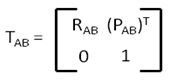Here A is the source frame and B is the target frame as shown in the figure.Therefore, including the last row [0 0 0 1], 3D transformation matrix is of size 4X4.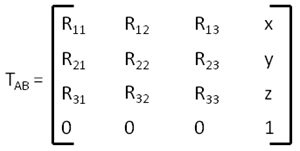Hurray....!!! Finally we have seen the 3D transformation matrix.

As explained in 2D transformation, 3D transformation matrix is used to find the transformation of nth frame w.r.t the base or 0th frame when only the intermediate transformations are known in 3D world.Fig. 15 Transformation of Frame n w.r.t Frame 0 using intermediate frames

Applications of 3D Transformations in robotics:

3D transformations are used in robotic arms, manipulators, humanoid robots, quadrotors, etc.

How to use these transformation matrices in robotics will be covered in Forward Kinematics. So see you there.